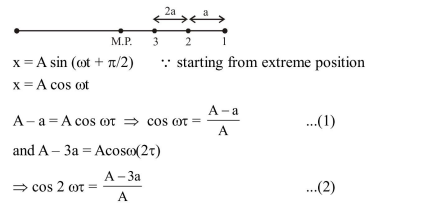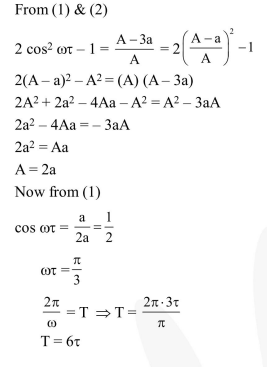# A particle moves with simple harmonic motion in a straight line.

Question:

A particle moves with simple harmonic motion in a straight line. In first $\tau \mathrm{s}$, after starting from rest it travels a distance a, and in next $\tau \mathrm{s}$ it travels $2 \mathrm{a}$, in same direction, then :

1. Amplitude of motion is 4 a

2. Time period of oscillation is $6 \tau$

3. Amplitude of motion is $3 \mathrm{a}$

4. Time period of oscillation is $8 \tau$

Correct Option: , 2

Solution: